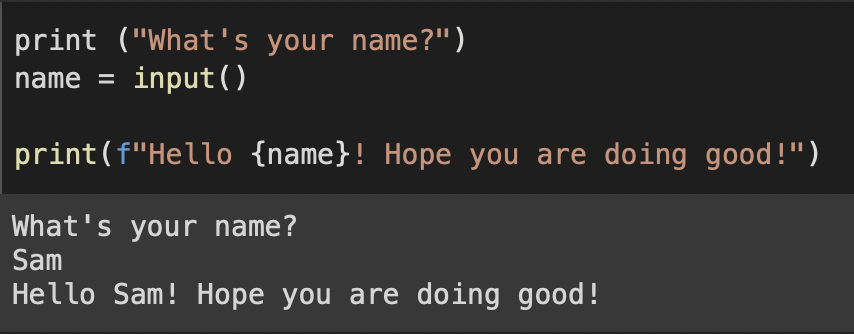# Take input argument from command line in Python ProgrammingIf you are a student learning Python Programming I am sure that your Teacher gives you a lot of code questions wherein you are asked to take inputs from the user from the command line or the Terminal to solve the problem.

In order to take input argument from the command line, you can make use of the input() function, let's see some examples,

Example 1: Take input from the command line as a name and display a greeting!
``````print ("What's your name?")
name = input()

print(f"Hello {name}! Hope you are doing good!")``````
Output:
``````What's your name?
Sam
Hello Sam! Hope you are doing good!``````
Example 2: Add two numbers by the inputs provided by the user as Terminal arguments
``````"""
In this example, we will
take a look at how to
get input arguments
from the user using the command line
in Python Programming

Author: Code2care.org
Date: 03-Apr-2022
Version: 1.0
"""

no1 = int(input())
no2 = int(input())

print ("Sum: ",no1+no2)``````
Output:
``````10
20
Sum:  30``````